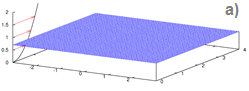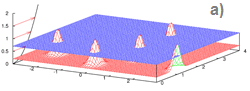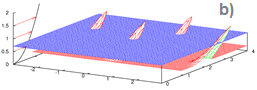# Two paradigms of streak formation in turbulent flowsFigure 1. A sketch of a visualisation plane parallel to the wall and the visualisation of streaks obtained in a numerical simulation.

Streaks are elongated regions where the instantaneous velocity is below average. If in the visualisation plane parallel to the wall (Figure 1a) one paints black the areas of small velocity, it will look like the picture in Figure 1b. Streaks are very important in turbulence. Why are they there? One thing is well established: areas of small velocity appear because there are wall-normal motions transporting slow-moving near-wall fluid away from the wall. This is called a lift-up mechanism. But why do the areas of small velocity have a streaky pattern?Figure 2. A sketch of longitudinal vortices and the schematics of streak induction by longitudinal vortices.

There are a least two possibilities. The answer that have been accepted for a long time was that the streaks have a pattern because the wall-normal motions have a pattern. Imagine, for example, a row of alternatively-rotating longitudinal vortices above a near-wall region of slow-moving fluid, as shown in Figure 2a. The curved surface shows an imaginary boundary of the slow-moving near-wall fluid. On one side of the vortex the fluid moves up, and on the other it moves down, thus forming alternating elongated stripes of upward and downward motions. The upward motions carry up the slow-moving fluid, and when it crosses the visualisation plane (Figure 2b) the streaks become visible. There are other patterns of wall-normal motion that also can induce streaks by the lift-up mechanism.Figure 3. Formation of streaks by a joint action of disordered wall-normal motion, means shear and diffusion.

It turns out, however, that streaks form even when the wall-normal motion has no pattern at all. Such wall-normal motion can be imposed in numerical experiments. Formation of streaks under such conditions requires a conceptually different explanation, and such explanation exists. It involves, however, not only lift-up, but also mean shear and diffusion. Indeed, consider the case when the wall-normal velocity is disordered and isotropic. Such upward motions alone will lead to patches in the visualisation plane having no pattern (Figure 3a). Shear will then tilt the peaks, shifting the picture in the visualisation plane, but not adding any pattern (Figure 3b). Then diffusion will smear this in wall-normal direction, and streaks will become visible (Figure 3c). Here, the pattern of streaks is dictated by the pattern-forming properties of combined lift-up, shear, and diffusion, rather than by the pattern of the wall-normal motion. Note that similar to how streaks can be created by different patterns of wall-normal motion, in the case of disordered wall-normal motion streaks might be formed by pattern-forming mechanisms different from that described above with Figure 3.

Which of these two concepts applies to the formation of streaks in near-wall turbulence? One hint follows from comparing the observed patterns of streaks and wall-normal motions. Observation shows that the characteristic spanwise scale of the longitudinal velocity, that is the average spanwise distance between streaks, is about 100 wall units, while the same quantity for the wall-normal velocity is about 50 wall units. This mismatch suggests that the second concept might be more close to the truth. Further confirmation follows from the extensive comparisons of quantitative predictions made on the basis of the second concept. In particular, the comparisons cover the dependence of streak spacing on the distance to the wall for velocity streaks, the dependence of the streak spacing on the mean profile of a passive admixture streaks, and the dependence of the streak angle on time in the flow past oscillating wall in the regime with drag reduction.

Developing quantitative prediction tools on the basis of the second concept requires resolving two important issues.

First, the pattern-forming mechanism of Figure 3 is linear. Turbulence is a nonlinear phenomenon. Hence, one needs to understand which of the known reasons for applicability of linearised approach to a nonlinear system is at work here. It appears that the first published reference for this reason was made by Lee, Kim and Moin in a paper published in JFM in 1990. They wrote: "the dominant mechanism in the production (and maintenance) of the preferred structures (i.e. streaks) in all turbulent shear flows at high shear rate is a selective amplification of eddies primarily by the linear interaction with mean shear". More recently this is referred to as the filtering property of the linearised Navier-Stokes equations.

Second, for making quantitative predictions it is necessary to recognise that in the case of the linearised Navier-Stokes equations the filtering property is strongly dependent on what is considered to be the "output", and that the right choice of the output is dictated by the quantity that is to be predicted by the analysis.

These two issues are discussed in a separate web page.